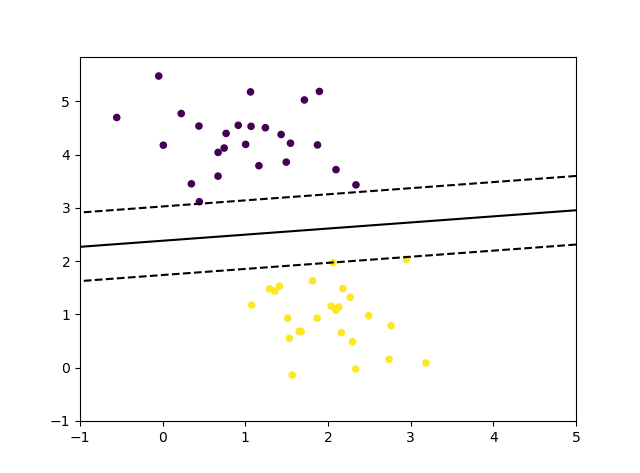# python机器学习库sklearn——SGD梯度下降法

python数据挖掘系列教程

Stochastic Gradient Descent （随机梯度下降法）的优势:

• 高效。
• 易于实现 (有大量优化代码的机会)。

Stochastic Gradient Descent （随机梯度下降法）的劣势:

• SGD 需要一些超参数，例如 regularization （正则化）参数和 number of iterations （迭代次数）。
• SGD 对 feature scaling （特征缩放）敏感。

## 随机梯度下降分类

loss function（损失函数） 可以通过 loss 参数来设置。 SGDClassifier 支持以下的 loss functions（损失函数）：

• loss=”hinge”: (soft-margin) linear Support Vector Machine （（软-间隔）线性支持向量机），
• loss=”modified_huber”: smoothed hinge loss （平滑的 hinge 损失），
• loss=”log”: logistic regression （logistic 回归），
• and all regression losses below（以及所有的回归损失）。

• penalty=”l2”: L2 norm penalty on coef_.
• penalty=”l1”: L1 norm penalty on coef_.
• penalty=”elasticnet”: Convex combination of L2 and L1（L2 型和 L1 型的凸组合）; (1 - l1_ratio) * L2 + l1_ratio * L1.

#===============随机梯度下降法分类===============

from sklearn.linear_model import SGDClassifier
from sklearn.datasets.samples_generator import make_blobs
import numpy as np
import matplotlib.pyplot as plt

X, y = make_blobs(n_samples=50, centers=2, random_state=0, cluster_std=0.60)
# loss：损失项。hinge：（软-间隔）线性支持向量机，modified_huber：平滑的 hinge 损失，log：logistic 回归，其他所有的回归损失
# penalty：惩罚项。l2：L2正则，l1：L1正则，elasticnet：(1 - l1_ratio) * L2 + l1_ratio * L1
clf = SGDClassifier(loss="hinge", alpha=0.01, max_iter=200, fit_intercept=True)  #
clf.fit(X, y)  # 训练模型

print('回归系数：',clf.coef_)
print('偏差：',clf.intercept_ )

# 绘制线，点
xx1 = np.linspace(-1, 5, 10)
xx2 = np.linspace(-1, 5, 10)

X1, X2 = np.meshgrid(xx1, xx2)  # X1、X2都是10*10的矩阵
Z = np.empty(X1.shape)
for (i, j), val in np.ndenumerate(X1):  # 迭代第i行第j列的坐标xx1取值为val
x1 = val
x2 = X2[i, j]  #
p = clf.decision_function([[x1, x2]])  # 计算输出值，也就是到超平面的符号距离。（支持向量到最佳超平面的符号距离为-1和+1）
Z[i, j] = p
levels = [-1.0, 0.0, 1.0]  # 将输出值分为-1,0,1几个区间
linestyles = ['dashed', 'solid', 'dashed']
plt.contour(X1, X2, Z, levels, colors='k', linestyles=linestyles)  # 绘制等高线图，高度为-1,0,1，也就是支持向量形成的线和最佳分割超平面
plt.scatter(X[:, 0], X[:, 1], c=y, s=20)  # 绘制样本点
plt.show()## 随机梯度下降法进行多分类

SGDClassifier 通过利用 “one versus all” （OVA）方法来组合多个二分类器，从而实现多分类。对于每一个 K 类, 可以训练一个二分类器来区分自身和其他 K-1 个类。

SGDClassifier 通过拟合参数 class_weight 和 sample_weight 来支持 weighted classes （加权类）和 weighted instances（加权实例）。

# ==============随机梯度下降法进行多分类=============
from sklearn.linear_model import SGDClassifier
from sklearn.metrics import accuracy_score
from sklearn import datasets
iris = datasets.load_iris()
X,y=iris.data,iris.target
clf = SGDClassifier(alpha=0.001, max_iter=100).fit(X, y)
y_pred = clf.predict(X)
print('三分类花卉数据准确率：',accuracy_score(y,y_pred))
print('包含的二分类器索引：',clf.classes_)    # one versus all 方法来组合多个二分类器
print('回归系数：',clf.coef_)  # 每一个二分类器的回归系数
print('偏差：',clf.intercept_ ) # 每一个二分类器的偏差

## 随机梯度下降法回归

SGDRegressor 类实现了一个简单的随机梯度下降学习例程，它支持用不同的损失函数和惩罚来拟合线性回归模型。 SGDRegressor 非常适用于有大量训练样本（>10.000)的回归问题，对于其他问题，我们推荐使用 Ridge ，Lasso ，或 ElasticNet 。

• loss=”squared_loss”: Ordinary least squares（普通最小二乘法）,
• loss=”huber”: Huber loss for robust regression（Huber回归）,
• loss=”epsilon_insensitive”: linear Support Vector Regression（线性支持向量回归）.

Huber 和 epsilon-insensitive 损失函数可用于 robust regression（鲁棒回归）。不敏感区域的宽度必须通过参数 epsilon 来设定。这个参数取决于目标变量的规模。

SGDRegressor 支持 ASGD（平均随机梯度下降） 作为 SGDClassifier。 均值化可以通过设置 average=True 来启用。

# #===============随机梯度下降法回归===============
from sklearn import linear_model
from sklearn.datasets import load_boston
X,y = load_boston().data,load_boston().target
clf = linear_model.SGDRegressor(loss='squared_loss',penalty='l2',alpha=0.01,max_iter=1000)
clf.fit(X, y)
print('得分：',clf.score(X,y))
print('回归系数：',clf.coef_)
print('偏差：',clf.intercept_ )